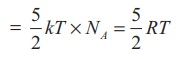Home | | Physics 11th std | Law of Equipartition of Energy

# Law of Equipartition of Energy

According to kinetic theory, the average kinetic energy of system of molecules in thermal equilibrium at temperature T is uniformly distributed to all degrees of freedom (x or y or z directions of motion) so that each degree of freedom will get 1/2 kT of energy. This is called law of equipartition of energy.

LAW OF EQUIPARTITION OF ENERGY

We have seen in Section 9.2.1 that the average kinetic energy of a molecule moving in x direction isSimilarly, when the motion is in y direction,According to kinetic theory, the average kinetic energy of system of molecules in thermal equilibrium at temperature T is uniformly distributed to all degrees of freedom (x or y or z directions of motion) so that each degree of freedom will get 1/2 kT of energy. This is called law of equipartition of energy.

Average kinetic energy of a monatomic molecule (with f=3) =Average kinetic energy of diatomic molecule at low temperature (with f = 5)Average kinetic energy of a diatomic molecule at high temperature (with f =7)Average kinetic energy of linear triatomic molecule (with f = 7) =Average kinetic energy of nonlinear tri atomic molecule (with f = 6) =## Application of law of equipartition energy in specific heat of a gas

Meyer’s relation CP CV = R connects the two specific heats for one mole of an ideal gas.

Equipartition law of energy is used to calculate the value of CP CV and the ratio between them γ = CP / CV.

Here γ is called adiabatic exponent.

### i)  Monatomic molecule

Average kinetic energy of a moleculeFor one mole, the molar specific heat at constant volume### ii)  Diatomic molecule

Average kinetic energy of a diatomic molecule at low temperature = 5/2kT

Total energy of one mole of gas(Here, the total energy is purely kinetic)

For one mole Specific heat at constant volumeEnergy of a diatomic molecule at high temperature is equal to 7/2RTNote that the CV and CP are higher for diatomic molecules than the mono atomic molecules. It implies that to increase the temperature of diatomic gas molecules by 1°C it require more heat energy than monoatomic molecules.### a)  Linear molecule### b)  Non-linear moleculeNote that according to kinetic theory model of gases the specific heat capacity at constant volume and constant pressure are independent of temperature. But in reality it is not sure. The specific heat capacity varies with the temperature.

### EXAMPLE 9.5

Find the adiabatic exponent γ for mixture of μ 1 moles of monoatomic gas and μ2 moles of a diatomic gas at normal temperature.

### SolutionTags : Kinetic Theory of Gases , 11th Physics : Kinetic Theory of Gases
Study Material, Lecturing Notes, Assignment, Reference, Wiki description explanation, brief detail
11th Physics : Kinetic Theory of Gases : Law of Equipartition of Energy | Kinetic Theory of Gases

Related Topics